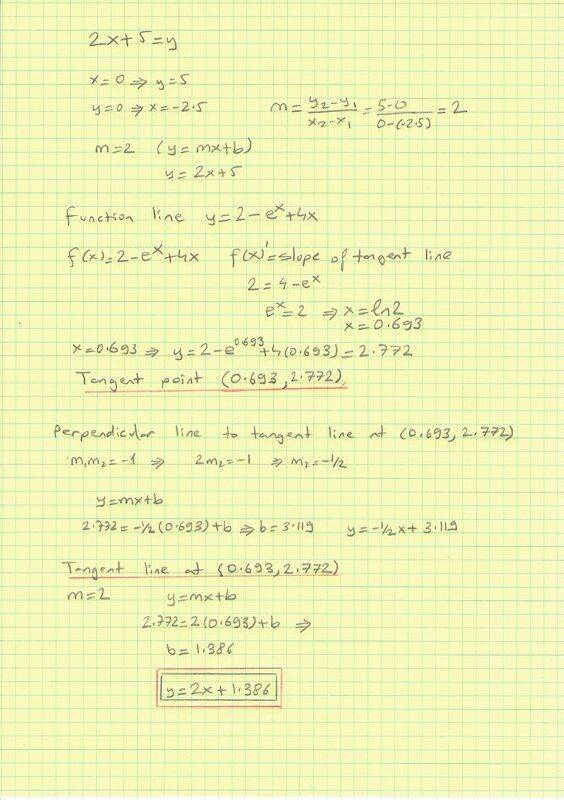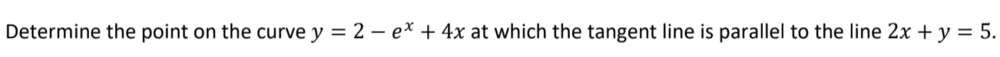# Determine the point on the given curve

• ttpp1124

#### ttpp1124

Homework Statement
I have my answer in an equation, but since it asks for a point, should I simply leave it as b=1.386?
Relevant Equations
n/aHomework Statement:: I have my answer in an equation, but since it asks for a point, should I simply leave it as b=1.386?
Relevant Equations:: n/a

View attachment 262591View attachment 262592
A point (in the context of this problem) has two coordinates, so the answer should be in the form of (x, y).

A point (in the context of this problem) has two coordinates, so the answer should be in the form of (x, y).
So I'd leave my final answer as (0.693,2.772)?

Homework Statement:: I have my answer in an equation, but since it asks for a point, should I simply leave it as b=1.386?
Relevant Equations:: n/a

View attachment 262591View attachment 262592
You have a mistake right off the bat. 2x + y = 5 is not equivalent to y = 2x + 5.

Instead of solving for the intercepts of the line and doing the slope formula, just rewrite the equation of the line in the form ##y=mx+b## then the coefficient ##m## of ##x## is the slope.